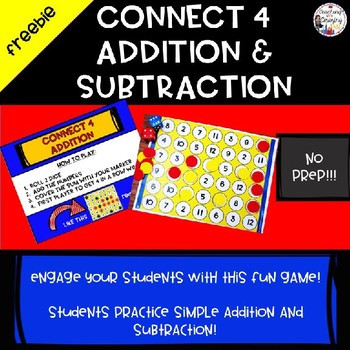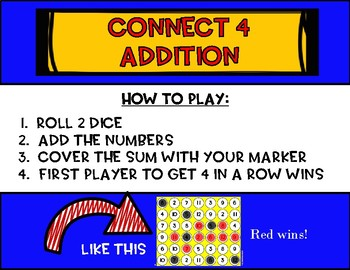# FREEBIE!! Connect Four Addition and Subtraction Fact PracticeSubject
Resource Type
File Type

PDF

(1 MB|11 pages)
Product Rating
Standards
• Product Description
• StandardsNEW
Connect 4: Addition and Subtraction - FREEBIE

This NO PREP set has FUN and engaging activities that meet Common Core Standards and TEKS Standards. Included are NO PREP activities and recording sheets (just print and go). Color and black/white game boards are included! The activities are perfect for math centers, morning work, homework folders, early finishers or small group instruction.

Connect 4: Addition and Subtraction is so easy to play. Students roll 2 dice and either add or subtract and cover the answer. The first player to have 4 in a row is the winner!!

Included in this set:
• 1 Color Connect 4 Addition
• 1 Black/White Connect 4 Addition
• 1 Color Connect 4 Subtraction
• 1 Black/White Connect 4 Subtraction
• 1 Black/White Recording Sheet

• Apply basic fact strategies to add within 10, including making 10 and decomposing a number leading to 10
• Understand that the equal sign represents a relationship where expressions on each side of the equal sign represent the same values
• Apply properties of operation to add two or three numbers

If you loved these, you can get more boards to put in your centers here!! Connect Four: Addition and Subtraction Fact Practice. The paid set includes 5 game boards for addition and 5 for subtraction AND a blank game board that you can use to make your own Connect 4 game.

Enrichment idea: Use the blank game board to have your students create their own game board. Have the students share with other students and play others games!

TEKS:
1.3C, 1.3D, 1.5E, 1.5F, 1.5G
Common Core:
1.OA.B.3, 1.OA.C.6, 1.OA.D.7, 1.OA.D.8, 1.NBT.C.4

Did you like this activity? Check out my store! Click the green star to follow and you will get email updates when I upload new products!!
Determine the unknown whole number in an addition or subtraction equation relating three whole numbers. For example, determine the unknown number that makes the equation true in each of the equations 8 + ? = 11, 5 = ▯ - 3, 6 + 6 = ▯.
Understand the meaning of the equal sign, and determine if equations involving addition and subtraction are true or false. For example, which of the following equations are true and which are false? 6 = 6, 7 = 8 - 1, 5 + 2 = 2 + 5, 4 + 1 = 5 + 2.
Add and subtract within 20, demonstrating fluency for addition and subtraction within 10. Use strategies such as counting on; making ten (e.g., 8 + 6 = 8 + 2 + 4 = 10 + 4 = 14); decomposing a number leading to a ten (e.g., 13 - 4 = 13 - 3 - 1 = 10 - 1 = 9); using the relationship between addition and subtraction (e.g., knowing that 8 + 4 = 12, one knows 12 - 8 = 4); and creating equivalent but easier or known sums (e.g., adding 6 + 7 by creating the known equivalent 6 + 6 + 1 = 12 + 1 = 13).
Apply properties of operations as strategies to add and subtract. If 8 + 3 = 11 is known, then 3 + 8 = 11 is also known. (Commutative property of addition.) To add 2 + 6 + 4, the second two numbers can be added to make a ten, so 2 + 6 + 4 = 2 + 10 = 12. (Associative property of addition.)
Add within 100, including adding a two-digit number and a one-digit number, and adding a two-digit number and a multiple of 10, using concrete models or drawings and strategies based on place value, properties of operations, and/or the relationship between addition and subtraction; relate the strategy to a written method and explain the reasoning used. Understand that in adding two-digit numbers, one adds tens and tens, ones and ones; and sometimes it is necessary to compose a ten.
Total Pages
11 pages
N/A
Teaching Duration
N/A
Report this Resource to TpT
Reported resources will be reviewed by our team. Report this resource to let us know if this resource violates TpT’s content guidelines.